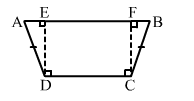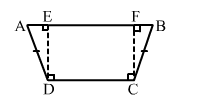# In the given figure, ABCD is a quadrilateral in which AD = BC and ∠ADC = ∠BCD.

Question:

In the given figure, ABCD is a quadrilateral in which AD = BC and ADC = ∠BCD. Show that the points ABCD lie on a circle.Solution:Draw DE ⊥ AB and CF ⊥ AB.
In ΔADE and ΔBCF, we have: